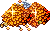## Swing Interval Calculator

Playguides written by Staff and Players.
Much more information on specific skills can be found in our T2A wiki.
Mikel123
UOSA Subscriber!Posts: 4607
Joined: Wed Jun 24, 2009 7:44 pm

### Swing Interval Calculator

This is going to be a short and sweet guide.

Calculating swing speed is kind of arduous and complex. It's easy to calculate in a spreadsheet program (like Excel) though. Here's the formula:

Code: Select all

``=MIN(MAX((ROUNDDOWN(60000/((STAM+100)*SPEED),0))*0.25,1.25),10)``
...where STAM is your current stamina, and SPEED is the weapon's speed (according to this table). You can simply remove those two variables and reference a cell in the sheet instead. For example:

Code: Select all

``=MIN(MAX((ROUNDDOWN(60000/((A2+100)*B2),0))*0.25,1.25),10)``
...where A2 has the stamina and B2 has the weapon speed you want to model.

What does this formula do? In words:

Take 60000 and divide by (STAM+100)*SPEED. This is the number of "ticks" between swings. Now lop off the decimal (in other words, round down to the nearest number of ticks). Multiply by 0.25 to put it into seconds (there's 4 ticks per second in UO time). This is your swing interval, which can never be less than 1.25 seconds and never more than 10 seconds.

mxmx23
Posts: 273
Joined: Wed Dec 24, 2008 4:19 pm

### Re: Swing Interval Calculator

Why do I read posts like this so early in the morning?

Now my brain hurts.